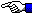# JDK 1.1 BigInteger

``` M. Gallant 10/14/97 ```

``` JDK1.1 includes two new classes for facilitating mathematical computation with arbitrary-precision numbers: BigInteger and BigDecimal. For further details, see the JDK1.1 Documentation. The example below shows a simple consecutive big-number multiplication example implemented in a Java application. The user enters two arbitrary integers at the command prompt. The integers are converted to BigInteger objects, multiplied and the result is squared 5 consecutive times. Note that BigIntegers are automatically made as large as necessary to accomodate calculation results. Note also that the implied toString() conversion method is supported as expected for the string concatenation operator in the println method. Java applications like this can conveniently be launched from a DOS shortcut. DOS System Output Java Source Code Java 1.1 Executable Bytecode import java.math.BigInteger; import java.io.* ; public class bigintest { public static void main(String args[]) throws IOException{ String I1, I2 ; BigInteger b1, b2, b3 ; PrintWriter dos = new PrintWriter(System.out, true) ; System.out.println("\n\n") ; System.out.println(" *************************************************"); System.out.println(" * ------------------------------------ *"); System.out.println(" * BigInteger Consecutive Multiplicaton *"); System.out.println(" * ------------------------------------ *"); System.out.println(" * *"); System.out.println(" * by M. Gallant 10/14/97 *"); System.out.println(" *************************************************"); System.out.println("\n\n"); BufferedReader disys = new BufferedReader(new InputStreamReader(System.in)); System.out.println("Enter first integer (I1):") ; I1=disys.readLine() ; System.out.println("\nEnter second integer (I2):") ; I2=disys.readLine() ; b1 = new BigInteger(I1) ; b2 = new BigInteger(I2) ; b3 = b1.multiply(b2) ; System.out.println("\n\n(I1 X I2) = " + b3) ; b3=b3.multiply(b3) ; // Square the previous result. System.out.println("\n\n(I1 X I2)^2 = " + b3) ; b3=b3.multiply(b3) ; // Square the previous result. System.out.println("\n\n(I1 X I2)^4 = " + b3) ; b3=b3.multiply(b3) ; // Square the previous result. System.out.println("\n\n(I1 X I2)^8 = " + b3) ; b3=b3.multiply(b3) ; // Square the previous result. System.out.println("\n\n(I1 X I2)^16 = " + b3) ; b3=b3.multiply(b3) ; // Square the previous result. System.out.println("\n\n(I1 X I2)^32 = " + b3) ; disys.close() ; } }Go Home ET ```